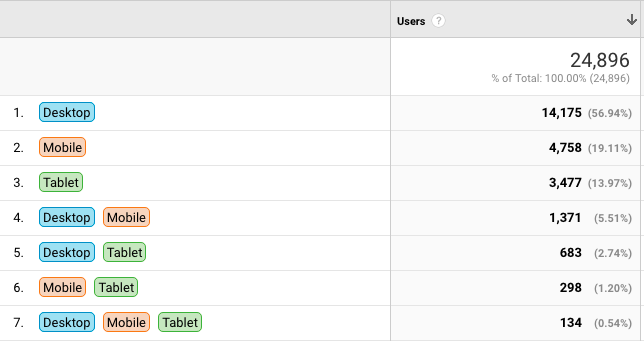Hi,

In Google Analytics, you have a venn diagram and a table showing cross device usage (for user id views). This is an example:I have another data set where I want to create a similar table, but I have no clue on how to do the calculation (Klipfolio or external). Does anyone have an idea on how to do it?

-Jørn

#### 1 comment

•Janice Janczyn

Hi Jørn,

You can either define your own groupings (in column deviceType)  and then use a LOOKUP to count the number of items using the various combinations of device types:

LOOKUP( !deviceType,
GROUP( GROUPBY( userIDs, devTypes, "join(values)" ) ),
COUNTDISTINCT( GROUPBY( userIDs, devTypes, "join(values)" ) ) )

Or you can define the device type groupings from the data:

GROUP( GROUPBY( userIDs, devTypes, "join(values)" ) )

and then count the number of items using each device type combination:

COUNTDISTINCT( GROUPBY( userIDs, devTypes, "join(values)" ) )

Thanks,
Janice